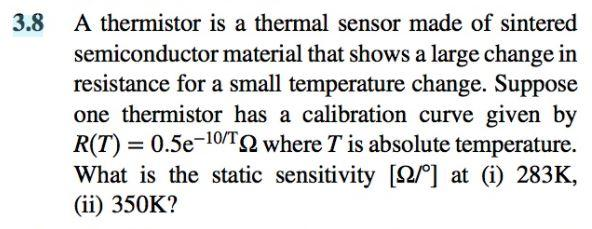# Question Solved1 Answer3.8 A thermistor is a thermal sensor made of sintered semiconductor material that shows a large change in resistance for a small temperature change. Suppose one thermistor has a calibration curve given by R(T) = 0.5e-10/T2 where T is absolute temperature. What is the static sensitivity [52/º] at (i) 283K, (ii) 350K?MEOMKC The Asker · Mechanical EngineeringTranscribed Image Text: 3.8 A thermistor is a thermal sensor made of sintered semiconductor material that shows a large change in resistance for a small temperature change. Suppose one thermistor has a calibration curve given by R(T) = 0.5e-10/T2 where T is absolute temperature. What is the static sensitivity [52/º] at (i) 283K, (ii) 350K?
More
Transcribed Image Text: 3.8 A thermistor is a thermal sensor made of sintered semiconductor material that shows a large change in resistance for a small temperature change. Suppose one thermistor has a calibration curve given by R(T) = 0.5e-10/T2 where T is absolute temperature. What is the static sensitivity [52/º] at (i) 283K, (ii) 350K?